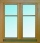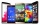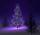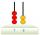A clock

A clock was set right at 6:00 AM. If it gains 3 1/2 minutes per hour, what time will it show at 6:00 PM on the same day? Show your solution

Result

t = 6:42 PMSolution:Leave us a comment of this math problem and its solution (i.e. if it is still somewhat unclear...):Be the first to comment!Next similar math problems:

1. Delayed clockMichael put a new battery into his watch at midnight. However, they are 5 seconds late each minute. How many hours does the watch show in 24 hours?After two hours and 40 minutes the job is finished. Compared to last year we were 40 minutes faster. What is the percentage increase to our performance?
3. Train delayThe train should be in the station for 10 minutes. It still has 32 km left. What is the expected delay if the train travels in 2 minutes 3 km except for the last section where it is 2 km in 5 minutes.
4. Mail trainThe speed of mail train is 1370 meter per minute. Express it in miles per hour correct to three significant figure . Given that 1 meter =39.37 inches.
5. Workers 9If two workers fix two windows in an hour how many windows can 18 workers fix in the same two windows?
6. Phone planVictoria's cell phone plan costs \$30.00 a month. If she used 12.5 hours in May, how much did Victoria pay per minute?
7. Christmas DayIn leap years was 53 Sundays. On what day of the week fell to Christmas Day?
8. Two agesGabrielle is 9 years younger than Mikhail. The sum of their ages is 87. What is Mikhail's age?
9. Masons1 mason casts 30.8 meters square in 8 hours. How long casts 4 masons 178 meters square?
10. Temperature conversionThe normal human body temperature is 98.6 degrees Fahrenheit. What is the temperature in degrees Celcius?
11. Greg and BillGreg is 18 years old. He is 6 less than 4 times Bill's age. How old is Bill?
12. Grandfather and grandmotherThe old mother is 5 years younger than the old father. Together they are 153 years old. How many years has each of them?
13. Simplify 2Simplify expression: 5ab-7+3ba-9
14. Simple equationsSolve system of equations: 5x+3y=5 5x+7y=25
15. FactoryIn the factory workers work in three shifts. In the first inning operates half of all employees in the second inning and a third in the third inning 200 employees. How many employees work at the factory?
16. Feet to milesA student runs 2640 feet. If the student runs an additional 7920 feet, how many total miles does the student run?
17. Degrees to radiansConvert magnitude of the angle α = 9°39'15" to radians: